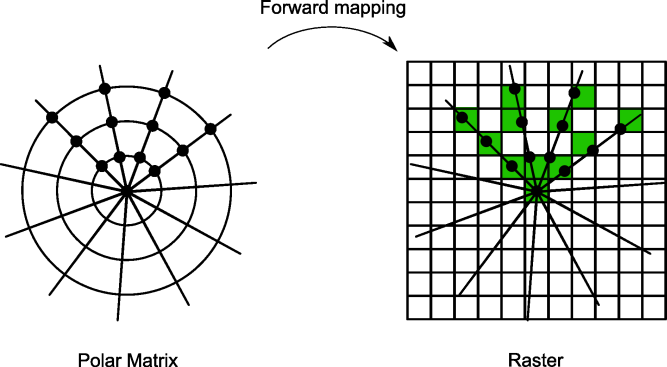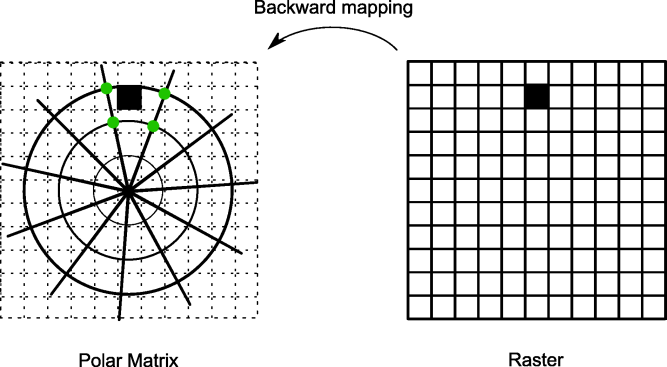2023.0.08

## Terrain Analysis EngineInterface ILcdMatrixRasterValueMapper

• `public interface ILcdMatrixRasterValueMapper`
Defines the requirements for an object that maps `double` matrix values to `short` raster values.

This interface is used to create a line-of-sight raster from a created line-of-sight matrix. Depending on the propagation function that was used to create the coverage matrix, different special values can be set to the matrix. In general, these special values should be handled in a different manner than normal values. For example, when creating a raster with fill mode `TLcdCoverageFillMode.MINIMUM`, the comparison between a special and normal value does not necessarily mean that the actual minimum should be used as value in the raster. This interface adds a way to define which value that should be used when comparing a matrix value with the current raster value.

There are two mapping possibilities. The first is called forward mapping and is done by using the method `mapMatrixValue`. Each matrix entry is mapped onto a raster pixel. When a raster pixel already contains a value, the method will determine the new value for that pixel using the given fill mode. Only, the modes `TLcdCoverageFillMode.MINIMUM` and `TLcdCoverageFillMode.MAXIMUM` are supported for this method. The following image clarifies this mapping technique:The second mapping approach is called backward mapping. Each raster pixel is mapped to a number of matrix entries. The values of these entries are combined to compute the new raster value. For this mapping technique, the method `combineMatrixValues` is used in combination with the fill mode `TLcdCoverageFillMode.NEAREST_NEIGHBOR`. The following image clarifies this:Note that this interface handles the matrix values independent from the associated points.

• ### Method Summary

All Methods
Modifier and Type Method and Description
`short` ```combineMatrixValues(double[] aMatrixValues, double[] aWeightsForMatrixValues, TLcdCoverageFillMode aFillMode)```
Combines matrix values depending on the given weights.
`short` `getDefaultValue()`
Returns the default raster value of this instance.
`short` ```mapMatrixValue(short aOldRasterValue, double aMatrixValue, TLcdCoverageFillMode aFillMode)```
Returns a raster value that is the result of a comparison between the given matrix value and the current raster value for a raster pixel.
• ### Method Detail

• #### combineMatrixValues

```short combineMatrixValues(double[] aMatrixValues,
double[] aWeightsForMatrixValues,
TLcdCoverageFillMode aFillMode)```
Combines matrix values depending on the given weights. Generally, the values are multiplied with the corresponding weight and the sum of those products is divided by the sum of all weights. This method should only do something else when one of the matrix values is a special value.

Currently, this method will only be used in combination with the nearest neighbor fill mode `TLcdCoverageFillMode.NEAREST_NEIGHBOR`. In this mode, the matrix value array contains only one value, the value corresponding to the matrix entry closest to the raster pixel. The weight array should not be used, because there is only one matrix value.

Parameters:
`aMatrixValues` - The matrix values to combine.
`aWeightsForMatrixValues` - The weights of the matrix values.
`aFillMode` - The fill mode to use.
Returns:
a raster value resulting from the combination of the given matrix values.
Throws:
`IllegalArgumentException` - if one of the matrix values is an unexpected value or if the given fill mode is not supported.
• #### mapMatrixValue

```short mapMatrixValue(short aOldRasterValue,
double aMatrixValue,
TLcdCoverageFillMode aFillMode)```
Returns a raster value that is the result of a comparison between the given matrix value and the current raster value for a raster pixel. The fill mode defines how the two values should be compared.
Parameters:
`aOldRasterValue` - The old raster value to compare to.
`aMatrixValue` - The matrix value to check.
`aFillMode` - The fill mode to use.
Returns:
a raster value that is the result of a comparison between the given matrix value and the current raster value for a raster pixel.
Throws:
`IllegalArgumentException` - if one of the matrix values is an unexpected value or if the given fill mode is not supported.
• #### getDefaultValue

`short getDefaultValue()`
Returns the default raster value of this instance. In general, this value corresponds to a transparent raster value.
Returns:
the default raster value of this instance.